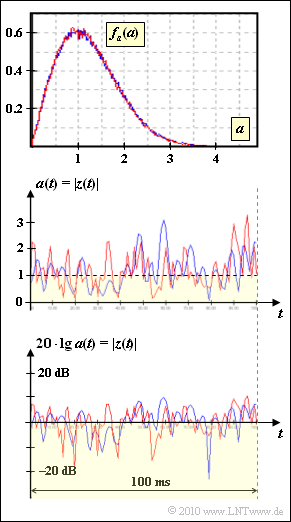# Exercise 1.4: Rayleigh PDF and Jakes PDSPDF and  $|z(t)|$  for Rayleigh fading with Doppler effect

We consider two different mobile radio channels with  Rayleigh fading. In both cases the PDF of the magnitude  $a(t) = |z(t)| ≥ 0$  is

$$f_a(a) = \frac{a}{\sigma^2} \cdot {\rm e}^{ -{a^2}/(2\sigma^2)} \hspace{0.05cm}.$$

The probability that this amount is not greater than a given value  $A$  is

$${\rm Pr}(|z(t)| \le A) = 1 - {\rm e}^{ -{A^2}/(2\sigma^2)} \hspace{0.05cm}.$$

The two channels, which are designated according to the colors "Red" and "Blue" in the graphs with  $\rm R$  and  $\rm B$  respectively, differ in the speed  $v$  and thus in the form of the power density spectrum  $\rm (PDS)$   ${\it \Phi}_z(f_{\rm D})$.

• For a Doppler frequency  $f_{\rm D}$  with  $|f_{\rm D}| <f_{\rm D,\hspace{0.1cm}max}$  the Jakes spectrum is given by
$${\it \Phi}_z(f_{\rm D}) = \frac{1}{\pi \hspace{-0.05cm}\cdot \hspace{-0.05cm}f_{\rm D, \hspace{0.1cm} max} \hspace{-0.05cm}\cdot \hspace{-0.05cm}\sqrt{ 1 \hspace{-0.05cm}- \hspace{-0.05cm}(f_{\rm D}/f_{\rm D, \hspace{0.1cm} max})^2} } \hspace{0.05cm}.$$
• For Doppler frequencies outside this interval from  $-f_{\rm D,\hspace{0.1cm}max}$  to  $+f_{\rm D,\hspace{0.1cm}max}$,   we have ${\it \Phi}_z(f_{\rm D})=0$.

The corresponding descriptor in the time domain is the autocorrelation function  $\rm (ACF)$:

$$\varphi_z ({\rm \delta}t) = 2 \sigma^2 \cdot {\rm J_0}(2\pi \cdot f_{\rm D, \hspace{0.1cm} max} \cdot {\rm \delta}t)\hspace{0.05cm}.$$
• Here,  ${\rm J_0}(.)$  is the Bessel function of the first kind and zeroth order.  We have  ${\rm J_0}(0) = 1$.
• The maximum Doppler frequency of the channel model  $\rm R$  is known to be   $f_{\rm D,\hspace{0.1cm}max} = 200 \ \rm Hz$.
• It is also known that the speeds  $v_{\rm R}$  and  $v_{\rm B}$  differ by the factor  $2$ .
• Whether  $v_{\rm R}$  is twice as large as  $v_{\rm B}$  or vice versa, you should decide based on the above graphs.

Notes:

### Questionns

1

Determine the Rayleigh parameter  $\sigma$  for the channels  $\rm R$  and  $\rm B$.

 $\sigma_{\rm R} \ = \$ $\ \ \rm$ $\sigma_{\rm B} \ = \$ $\ \ \rm$

2

In each case, give the probability that  $20 \cdot {\rm lg} \ a ≤ -10 \ \ \ \rm dB$   ⇒   $a ≤ 0.316$.

 Channel  ${\rm R}\text{:} \hspace{0.4cm} {\rm Pr}(a ≤ 0.316) \ = \$ $\ \rm \%$ Channel  ${\rm B}\text{:} \hspace{0.4cm} {\rm Pr}(a ≤ 0.316) \ = \$ $\ \rm \%$

3

Which statements are correct regarding the driving speeds  $v$ ?

 $v_{\rm B}$  is twice as big as  $v_{\rm R}$. $v_{\rm B}$  is half as big as  $v_{\rm R}$. With  $v = 0$,   $|z(t)|$  would be constant. With  $v = 0$,   $|z(t)|$  would have a white spectrum. With  $v → ∞$,   $|z(t)|$  would be constant. With  $v → ∞$,   $|z(t)|$  would be white.

4

Which of the following statements are correct?

 The PDS value  ${\it \Phi_z}(f_{\rm D} = 0)$  is the same for both channels. The ACF value  $\varphi_z(\Delta t = 0)$  is the same for both channels. The area under  ${\it \Phi_z}(f_{\rm D})$  is the same for both channels. The area below  $\varphi_z(\Delta t)$  is the same for both channels.

### Solution

#### Solution

(1)  The maximum value of the PDF for both channels is  $0.6$  and occurs at  $a = 1$.

• The Rayleigh PDF and its derivative are
$$f_a(a) \hspace{-0.1cm} = \hspace{-0.1cm} \frac{a}{\sigma^2} \cdot {\rm e}^{ -a^2/(2\sigma^2)} \hspace{0.05cm},$$
$$\frac{{\rm d}f_a(a)}{{\rm d}a} \hspace{-0.1cm} = \hspace{-0.1cm} \frac{1}{\sigma^2} \cdot {\rm e}^{ -a^2/(2\sigma^2)}- \frac{a^2}{\sigma^4} \cdot {\rm e}^{ -a^2/(2\sigma^2)} \hspace{0.05cm}.$$
• By setting the derivative to  $0$, you can show that the maximum of the PDF occurs at  $a = \sigma$.  Since the Rayleigh PDF applies to both channels, it follows that
$$\sigma_{\rm R} = \sigma_{\rm B} \hspace{0.15cm} \underline{ = 1} \hspace{0.05cm}.$$

(2)  As they fading coefficients have the same PDF, the desired probability is also the same for both channels.

• Using the given equation, we have
$${\rm Pr}(a \le 0.316) = {\rm Pr}(20 \cdot {\rm lg}\hspace{0.15cm} a \le -10\,\,{\rm dB}) = 1 - {\rm e}^{ -{0.316^2}/(2\sigma^2)} = 1- 0.951 \hspace{0.15cm} \underline{ \approx 4.9 \%} \hspace{0.05cm}.$$

(3)  The correct statements are 2, 3 and 6:

• The smaller speed  $v_{\rm B}$  can be recognized by the fact that the magnitude  $|z(t)|$  changes more slowly with the blue curve.
• When the vehicle is stationary, the PDS degenerates to  ${\it \Phi_z}(f_{\rm D}) = 2\sigma^2\cdot \delta(f_{\rm D})$,  and we have  $|z(t)| = A = \rm const.$, where the constant  $A$  is drawn from the Rayleigh distribution.
• At extremely high speed, the Jakes spectrum becomes flat and has an increasingly small magnitude over an increasingly wide range.  It then approaches the PDS of white noise.  However,  $v$  would have to be in the order of the speed of light.

(4)  Statements 2 and 3 are correct:

• The Rayleigh parameter  $\sigma = 1$  also determines the "power"  ${\rm E}[|z(t)|^2] = 2\sigma^2 = 2$  of the random process.
• This applies to both  $\rm R$  and  $\rm B$:
$$\varphi_z ({\rm \delta}t = 0) = 2 \hspace{0.05cm}, \hspace{0.2cm} \int_{-\infty}^{+\infty}{\it \Phi}_z(f_{\rm D}) \hspace{0.15cm}{\rm d}f_{\rm D} = 2 \hspace{0.05cm}.$$